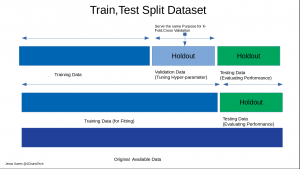# How to Split Dataset into Training and Testing Dataset For Machine Learning

One of the most important activities when building models is the ability to test and evaluate your model and have something to compare with that shows that your model actually worked.

In order to do this you will need to have enough data to train and build a working model and also to test the model you’ve built . So we approach this issue by splitting our initial data into two main parts which includes

• Training Data
• Testing Data

In certain cases we can split them into 3 parts which includes

• Training Data: used for building and fitting during training
• Testing Data: used for evaluating the performance of our model
• Validation Data: used for hyper-parameterization and tuning of model

Below is a picture that explains itLet us see how to split our dataset into training and testing data. We will be using 3 methods namely

• Using Sklearn train_test_split
• Using Pandas .sample()
• Using Numpy np..split()

Using Sklearn to Split Data – train_test_split()

To use this method you will have to import the train_test_split() function from sklearn and specify the required parameters.

The params include

test_size: how you want to split the test data by e.g. 0.30% which is  30% of the entire data will be the testing data

random_state: an integer that ensures that you randomly get the same subsets of the data-set every time.

stratified: function to ensure that both the train and test sets have the proportion of examples in each class that is present in the provided “y class

```from sklearn.model_selection import train_test_split

X_train, X_test, y_train, y_test = train_test_split(X, y, test_size=0.33, random_state=1)```

Using Pandas – df.sample()

You can also use pandas .sample() function and specify the fraction of data you want to fetch and then use the index of the fetched data as a guide to drop the rest. This is useful if your dataset is a dataframe.

``````train=df.sample(frac=0.8,random_state=200)
````test=df.drop(train.index)````

You may also want to split your data into features and the label part. We can do this by simply using the indexing approach or the long format of checking the columns and the labels and setting them as required.

`X, y = df[:, :-1], df[:, -1]`

Using Numpy np.split()

Numpy has a split() that allows you split arrays into partitions as you want. In our case we will be spliting our dataset using 67 percent of the length of the entire dataset (int(0.67 * len(df)) for our first part and the remaining as or testing dataset.

```# using numpy to split into 2 by 67% for training set and the remaining for the rest
train,test = np.split(df,[int(0.67 * len(df))])```

To conclude we have seen three basic methods to split our dataset into  training and testing data.